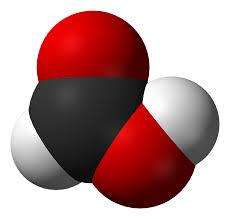# Concentration 3097

How many liters of 60% and 80% formic acid do we have to mix to get 80 liters of this acid with a concentration of 65%?

a =  60
b =  20

### Step-by-step explanation:

0.60a+0.80b = 0.65·80
a+b = 80

0.60·a+0.80·b = 0.65·80
a+b = 80

0.6a+0.8b = 52
a+b = 80

Pivot: Row 1 ↔ Row 2
a+b = 80
0.6a+0.8b = 52

Row 2 - 0.6 · Row 1 → Row 2
a+b = 80
0.2b = 4

b = 4/0.2 = 20
a = 80-b = 80-20 = 60

a = 60
b = 20

Our linear equations calculator calculates it.Did you find an error or inaccuracy? Feel free to write us. Thank you!

Tips for related online calculators
Do you have a system of equations and looking for calculator system of linear equations?
Tip: Our volume units converter will help you convert volume units.